4150.0 - Time Use Survey: User Guide, 2006
Latest ISSUE Released at 11:30 AM (CANBERRA TIME) 21/02/2008
Page tools:Print AllRSSSearch this Product
Contents >> Survey Methodology >> WEIGHTING, BENCHMARKING AND ESTIMATION

WEIGHTING, BENCHMARKING AND ESTIMATION

Weighting

'Weighting' is the process of adjusting results from a sample survey to infer results for the total population. To do this, a 'weight' is allocated to each sample unit, e.g. person-day, person or household. The weight is a value which indicates how many population units are represented by the sample unit.

The 2006 Time Use Survey was conducted over four 13-day collection periods and population distributions appropriate to each period were used in the weighting process. Significant changes in the weighting methodology from that used in the 1997 Time Use Survey have been instituted to:

• calculate more appropriate initial selection weights;
• better deal with differences in non-response and sampling fractions for each State and Territory by region; and
• utilise a finer level estimated distribution of the person and person-day populations.

Person-day weights

Person-day estimates obtained from the Time Use Survey were derived using a complex ratio estimation procedure. Estimates from the survey were obtained by weighting person-day responses to represent the in-scope population of the survey. Calculation of weights for person-days was carried out in two steps, the first being the calculation of the initial weight, and the second being the calibration to population benchmarks.

An initial weight was calculated for each person-day as the inverse of the person-day's probability of selection. The weight is a function of the probability of selection of the person with an adjustment applied for day type (weekday/Saturday/Sunday).

Households were selected for the survey by selecting CDs within each State by region (Capital City / Balance of State) stratum by a method known as probability proportional to size. The selected CDs were then systematically randomly allocated to collection periods such that each State by region had an equal number of CDs in each period. Blocks were then selected within CDs, and households within blocks. The selection method was designed to achieve, as far as practicable, a known and equal chance of selection for each household within each State by region stratum.

As CDs were allocated equally across collection periods, the probability of a CD and thus a household being selected in a particular period was not dependent upon the period. Therefore, the probability of a household being selected in any of the four enumeration periods was the same within each State by region stratum.

All in-scope persons in a selected household were surveyed, therefore, the probability a person was selected for the survey is the same as the probability their household was selected, and a person's initial weight is the same as their household's initial weight. Each selected person was asked to respond for two diary days, therefore the person level initial weight was divided by two to get the person-day unadjusted initial weight.

To ensure sufficient sample was collected for each day type (weekday/Saturday/Sunday), CDs (and thus households and persons) were assigned dates such that approximately 1/9 of the survey diary days were allocated to each of Monday, Tuesday, Wednesday, Thursday and Friday, and 2/9 of the survey diary days were allocated to each of Saturday and Sunday. However, the sample was to be weighted up to the in-scope person-day population such that weekdays would comprise 5/7 of the population, and Saturday and Sunday 1/7 each. Therefore, the final person-day initial weight was calculated by dividing the person-day unadjusted initial weight by the proportion of the day type assigned to the sample, and multiplied by the appropriate fraction for that day type.

The final person-day weights were determined by adjusting the initial weights so the estimated population distribution simultaneously conformed to two sets of population benchmarks.

Person weights and household weights

Person and household estimates obtained from the Time Use Survey were also derived using a complex ratio estimation procedure. Person and household estimates from the survey were obtained by weighting person level and household level responses, respectively, to represent the in-scope population of the survey. Calculation of weights for persons and households was carried out in two steps, the first being the calculation of the initial weight, and the second being the calibration to population and household benchmarks, respectively.

As all in-scope persons in a selected household were surveyed, a person's initial weight is equal to their household's initial weight. A household's probability of selection and initial weight, and therefore a person's initial weight was defined previously in the Person-day weight section.

The final person weights were determined by adjusting the initial weights so the estimated population distribution simultaneously conformed to two sets of population benchmarks. Similarly, the final household weights were determined by adjusting the initial weights so the estimated household distribution simultaneously conformed to two sets of resident household distribution benchmarks.

Benchmarking

For the person-day and person benchmarking, two sets of population distributions (benchmarks) were used for each collection period of the Time Use Survey. Similarly, for the household benchmarking, two sets of household benchmarks were used for each collection period.

Person-day benchmarks

The first set of person-day benchmarks was at the State by region (Capital City/Balance of State or Territory) by sex level and was obtained by averaging population distribution estimates on each side of the time use collection periods. The averaged values were then divided by four, so that the benchmarks for each collection period summed to a quarter of the in scope population for that period. Therefore, the sum of the benchmarks over all four collection periods is an estimate of the total in scope population for the 2006 survey.

The second set of benchmarks was at the sex by age group by employment status by day type level. These benchmarks were obtained by applying employment status proportion estimates (employed/not employed) to appropriate population estimates on each side of the Time Use Survey collection periods and then averaging the two resulting estimated population distributions for each period. Again, the averaged values were divided by four, so that the benchmarks for each collection period summed to a quarter of the in scope population for that period. Finally, the benchmarks were split by day type to ensure the final weighted sample would represent the appropriate population-day distribution. Each sex by age group by employment status population count was multiplied by 5/7, 1/7 and 1/7 to calculate the weekday, Saturday and Sunday benchmark estimates respectively.

The benchmarks used in the final weights for TUS 2006 were:

• number of persons aged 15 years and over -
• at State by region (Capital City/Balance of State or Territory) by sex
• at sex by age group by employment status by day type
• age groups are defined as 15-19, 20-24, 25-34, 35-44, 45-54, 55-64, 65-74, 75+
• employment status is defined as employed or unemployed (Note that employment status is not calculated for persons aged 65 years or older as the estimated proportion employed is small, with a large associated standard error. Therefore a third code is defined: aged 65 years or older)
• day type is defined as weekday/Saturday/Sunday

Person benchmarks

The first set of person level benchmarks are the same as those used for the person-day benchmarking. The second set of person benchmarks use sex by age group by employment status as for the second set of the person-day benchmarks, but without the split by day type.

Household benchmarks

The first set of benchmarks was at the State by region level, and the second at the household composition (number of adults and children) level. Both sets were obtained by averaging resident household estimates available for periods just before and just after each of the four 2006 Time Use Survey enumeration periods. These averages were then divided by four, so that the benchmarks for each collection period summed to a quarter of the in scope household population for that period. The sum of the benchmarks over all four collection periods is an estimate of the total population of households for the 2006 survey.

The benchmarks used in the final weights for TUS 2006 were:

• at state by region; and
• household composition.

Estimation

Person-day estimates

All person level time use estimates in the 2006 Time Use Survey publication were calculated from selected person-day weighted responses, using the weights described above. Formulae for five common types of estimates in this publication are:

1. the number of persons in classification c is estimated using: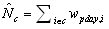where wpday,i is the final weight of the person-day and the summation is over all persons in classification c ;

2. the number of persons in classification c participating in an activity on an average day is estimated using: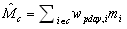where mi is 1 if a person participated in the activity or 0 if they didn't;

3. the total number of minutes spent on an activity by all persons in classification c on an average day is estimated using: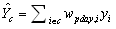where yi is the number of minutes spent by each person on the activity;

4. the average number of minutes spent by all persons (participant and non-participant) in classification c on an activity on an average day is estimated using: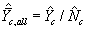5. the average number of minutes spent by participating persons only in classification c on an activity on an average day is estimated using: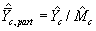Person estimates

Appropriate person level count and percentage estimates can be calculated from selected person weighted responses, using the weights described above. Note that you cannot calculate any time related estimates using these weights, as they are not applicable at the person-day level. Formulae for three common types of estimates are:

1. the number of persons in classification c is estimated using: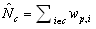where wp,i is the final weight of the person and the summation is over all persons in classification c;

2. the number of persons in classification c with some other attribute (e.g. self-description of own health, frequency of feeling pressed for time) is estimated using: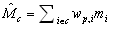where mi is 1 if a person holds the attribute of concern, or 0 if they don't; and

3. the proportion of persons in classification c with some other attribute is estimated using:Household estimates

All household level estimates in the 2006 Time Use Survey publication were calculated from selected household weighted responses, using the weights described above. Formulae for three types of estimates are:

1. the number of households in classification c is estimated using: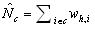where wh,i is the final weight of the household and the summation is over all households in classification c;

2. the number of households in classification c with a piece of equipment (e.g. microwave) or utilising a service (e.g. cleaner) is estimated using: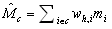where mi is 1 if a household had the piece of equipment or utilised the service, or 0 if they didn't; and

3. the proportion of households in classification c with a piece of equipment or utilising a service is estimated using: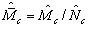Previous Page Next Page